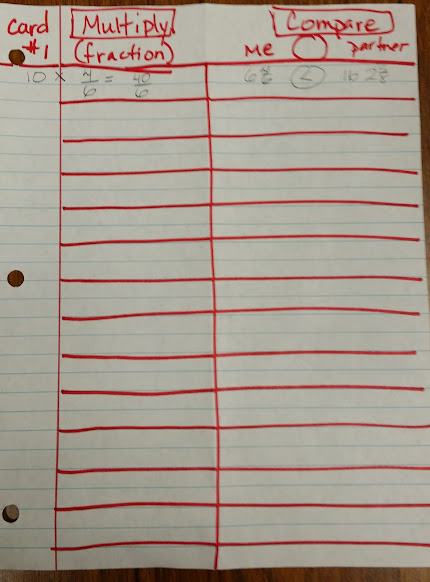# Fraction CARD GAME

11 teachers like this lesson
Print Lesson

## Objective

SWBAT practice multiplying a whole number with a fraction.

#### Big Idea

In this lesson, students work with partners to play a card game in which they can refine and practice the skill of multiplying a fraction with a whole number.

## Warm Up

5 minutes

For this warm up I present a fraction word problem to students.  This gives students an opportunity to apply skills learned in a previous lesson involving multiplying a whole number with a fraction.  I display this problem:

A recipe for brownies calls for 3/8 cup of milk. If Tina needs to make 3 batches, how much milk will she need?

I then give students about 7 minutes to complete the problem. When most students are done, I ask about 3 or 4 student volunteers to share their thinking using the document camera.

## Concept development

50 minutes

In this lesson, students work on Common Core standard .4.NF.B.4a  and .4.NF.B.4b .

Students work to understand a multiple of a/b as a multiple of 1/b, and use this understanding to multiply a fraction by a whole number. For example, use a visual fraction model to express 3 × (2/5) as 6 × (1/5), recognizing this product as 6/5. (In general, n × (a/b) = (n × a)/b.)

Students also work to understand a fraction a/b as a multiple of 1/b. For example, use a visual fraction model to represent 5/4 as the product 5 × (1/4), recording the conclusion by the equation 5/4 = 5 × (1/4)

I want my students to have opportunities to practice the skill of multiplying a fraction with a whole number in this lesson. This is an important skill to master for the above standards. In order to practice this I designed a card game for students to play.

Students play with their learning partner using a regular deck of playing cards.

I show students how to play this game and set up their papers.  You can see in the photo below an example of how students set up their paper for playing this game. By having my students use this structure, I have found that it helps keep them more focused and on task. It also ensures that students record each other's fractions and promotes team work.  While I have my students set up their papers this way, it is not necessary to play the game.To play:

1. Each students draws a card. This becomes their first card and becomes their whole number. My students record that number on the left  hand side of their paper.

2.  Next, each student takes turns and draws two more cards. The first card drawn becomes the numerator and the second card becomes the denominator. Again, students record their fractions on their paper.

3.  Then students multiply their whole number with the fraction they created and record that on their game sheet or paper.  Students record their product as a mixed number.  I tell students to use the rectangle below the multiplication problem to model and create a mixed number when applicable.

4.  Each partner records his/her problem and product on their own paper.  Then the partners record each others products in a comparison problem and write a greater than, less than, or equal sign.

While students play this game, I am circulating around the room listening to their strategies and asking questions to deepen their thinking.  I am observing which students are using an algorithm, and which students are using models to convert their improper fraction to a mixed number.

You can see and hear students playing this game in this video.  Notice in the beginning of the video the amount of thinking the girl student is doing. She is focused and on task.  This is one reason I particularly like to use games as instructional strategies.  Designing engaging games motivates students, provides productive struggle, and deepens their understanding.

You can see in this video a student attempting to model 42/12.  This student struggles some at figuring out how to model 42/12.  Listen in and see what kinds of questions I use with this student and the amount of wait time I use as he thinks through this problem.

## Student debrief - Wrap up

5 minutes

For this exit ticket students solve 7 x 4/6

I chose to use an exit ticket today in order to gain an understanding of which students are able to multiply a fraction with a whole number and which students still need more time with this skill. I also wanted to get a grasp on which strategies students are using to convert their improper fraction to a mixed number.

Note:  Upon analysis of the exit tickets from my first class of 27 students, 7 out of 27 calculated an incorrect answer while the others had a correct answer. This is roughly 25% of the class. I will small group these seven students to re-teach and review multiplying fractions with whole numbers.